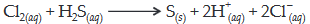#### Consider the reaction :The rate of reaction for this reaction isRate =Which of these mechanism is/are consistent with this rate equation ?Option 1) A only Option 2) B only Option 3) Both A and B Option 4) Neither A nor BAs we learnt in

Rate Determining Step -

The overall rate of reaction is controlled by the slowest step in a reaction called the rate determining step.

- wherein

e.g.For ReactionThe Mechanism isOption 1)

A only

This option is correct.

Option 2)

B only

This option is incorrect.

Option 3)

Both A and B

This option is incorrect.

Option 4)

Neither A nor B

This option is incorrect.

#### divya.saini